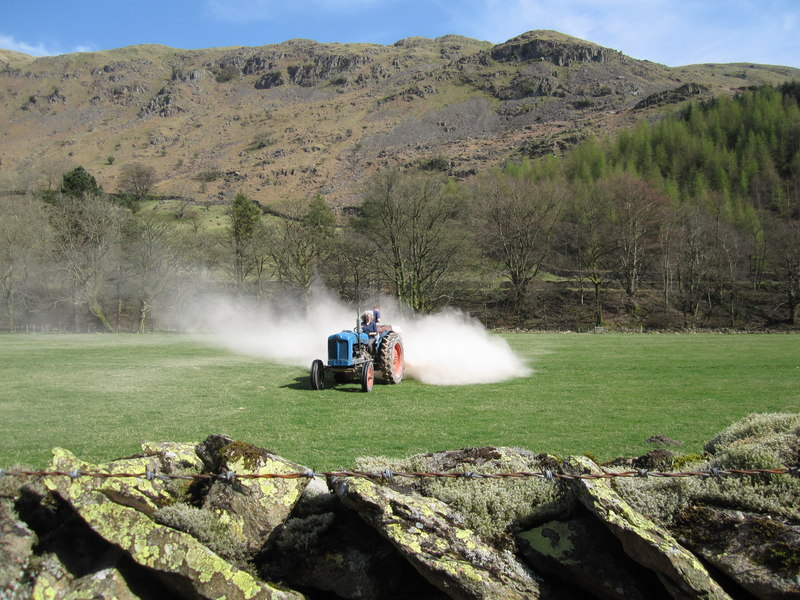1. Rain water is weakly acidic because it contains dissolved carbon dioxide. Which of these is a possible pH for rain water?

2. Litmus, phenolphthalein and methyl orange are all indicators which can be used to distinguish between acids and alkalis.

What are the correct colours of these indicators in alkali?

 litmus phenolphthalein Methyl orange A blue colourless yellow B blue pink yellow C blue pink red D red colourless red
3. Four solutions with different pH values were tested with universal indicator.

Which row shows the colour of each solution after the indicator was added?

 pH 9 pH 1 pH7 pH6 A purple red yellow orange B red yellow green blue C blue red green yellow D blue green yellow orange
4. Slaked lime (calcium hydroxide) is used to neutralize acidic soils.Which ion is responsible for neutralizing the acid in the soil?
• A.   Ca2+
• B.   H+
• C.   OH-
• D.   O2-
5. Which is NOT a typical characteristic of acids?
• A.   They react with metal oxides producing hydrogen
• B.   They react with carbonates producing carbon dioxide
• C.   They react with alkalis producing water
• D.   They turn blue litmus paper red

6. Acids form salts. Select the row that correctly states the type of salt formed from each acid.

 Hydrochloric acid Sulfuric acid Nitric acid A chlorate sulfide nitride B chlorate sulfate nitrate C chloride sulfate nitride D chloride sulfate nitrate

Q7-9:
A student prepared a sample of hydrated copper(II)sulfate crystals by reacting excess copper(II)carbonate with dilute sulfuric acid.7. The equation for this reaction with the correct state symbols is ..
• A.  CuCO3(s) + H2SO4(l)  CuSO4(l) + H2O(l) + CO2(g)
• B.  CuCO3(s) + H2SO4(aq)  CuSO4(aq) + H2O(l) + CO2(g)
• C.  CuCO3(s) + H2SO4(l)  CuSO4(aq) + H2O(l) + CO2(g)
• D.  CuCO3(aq) + H2SO4(aq)  CuSO4(aq) + H2O(l) + CO2(g)
8. How would the student know when she had added an excess of copper(II)carbonate?
• A.   the solution would turn blue in colour
• B.   the temperature would rise
• C.   effervescence would stop and solid would remain in the mixture
• D.   all the solid would disappear

9. What techniques would the student use to:

(a) separate the excess copper(II)carbonate from the mixture?
(b) obtain hydrated copper(II)sulfate crystals from the solution?

 Separate excess copper(II) carbonate Obtain hydrated copper(II)sulfate crystals A filtration crystallisation B evaporation distillation C filtration distillation D evaporation crystallisation
10. Which statement is NOT true about bases?
• A.   Bases are proton acceptors
• B.   An alkali is an insoluble base
• C.   Copper(II)hydroxide and ammonia are bases
• D.   Bases can neutralize acids